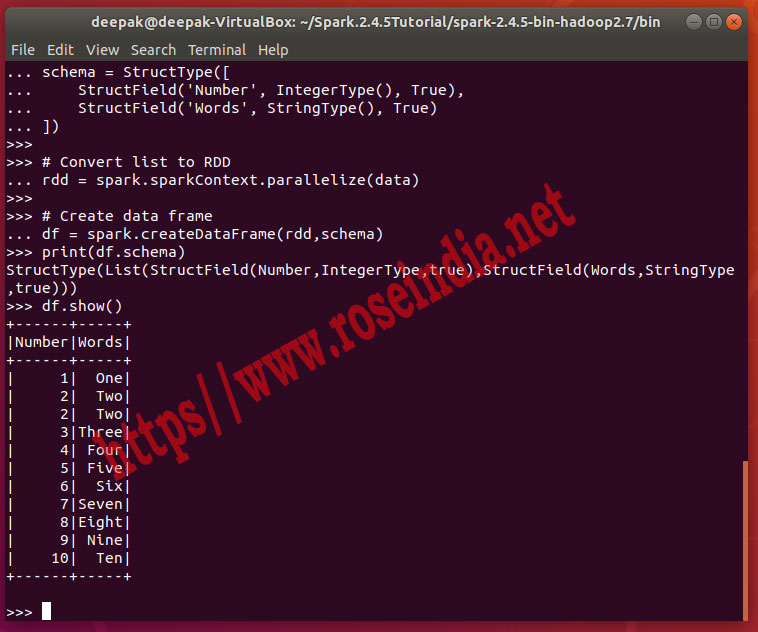Tutorials

# With PySpark read list into Data Frame

In this tutorial we are developing PySpark program for reading a list into Data Frame. You will be able to run this program from pyspark console and convert a list into Data Frame.

In this tutorial we are developing PySpark program for reading a list into Data Frame. You will be able to run this program from pyspark console and convert a list into Data Frame.

## Reading a list into Data Frame in PySpark program

The Spark SQL comes with extensive libraries for working with the different data sets in Apache Spark program. The SparkSQL is most used library in Spark framework and it is used to process large collection of data over distributed cluster. The preferred way is to convert the data into Data Frame before performing any action and transformations on the data set. In this tutorial we are going to develop a program in PySpark which converts a list (in Python) to the Spark Data Frame. If you don't know about Spark Data Frame and Data Structures of Spark then check our tutorial: Apache Spark data structures.

### What is Data Frame in Apache Spark?

The Data  Frame is Spark Data Structure which is again a distributed collection of data which can be processed over the spark distributed cluster. The Apache Spark Data Frame Data Structure stores the data into rows and columns. This data is processed by the SparkSQL library and enables the developers to perform sql like queries.

### Example of reading list and creating Data Frame

Now lets write some examples. For converting a list into Data Frame we will use the createDataFrame() function of Apache Spark API. The createDataFrame() function is used to   create data frame from RDD, a list or pandas DataFrame. This function come with flexibility to provide the schema while creating data frame.

Here is the syntax of the function:

`createDataFrame(data, schema=None, samplingRatio=None, verifySchema=True)`

So, with the help of this function we can create Creates a DataFrame object by using the existing RDD, a list or DataFrame of pandas.

Here is the list in Python that we would like to convert to the PySpark DataFrame:

``oneToTen = [1, 2, 3, 4, 5, 6, 7, 8, 9, 10]``

Here is one example which uses createDataFrame() and specifies the data type to be used for the column:

``````
from pyspark.sql.types import IntegerType

# Create List
oneToTen = [1, 2, 3, 4, 5, 6, 7, 8, 9, 10]

# Convert to Dataframe
df = spark.createDataFrame(oneToTen, IntegerType())

# Display data
df.show()
``````

In the above program we have provided list and the IntegerType() as parameter for creation of data frame. Here is the screen shot of the execution of the program on Spark:Here is another method of reading the list into Data Frame in PySpark (using Python):

``````
from pyspark.sql import Row

# Create List
oneToTen = [1, 2, 3, 4, 5, 6, 7, 8, 9, 10]

oneToTenRdd = sc.parallelize(oneToTen)
oneToTenRowRdd = oneToTenRdd.map(lambda x: Row(x))
df=sqlContext.createDataFrame(oneToTenRowRdd,['numbers'])

df.show()
``````

Above example uses the map and lambda functions for creating the RDD and then from RDD data frame is created. Here is the screen shot of the program executed on the Spark:There is third way of creating the Data Frame from list by providing the Data Frame schema. Here we have to first define the schema and use it with the createDataFrame() function to create Data Frame from rdd. Here is the complete example code:

``````
from pyspark.sql import SparkSession
from pyspark.sql.types import ArrayType, StructField, StructType, StringType, IntegerType

data = [(1, "One"),
(2, "Two"),
(2, "Two"),
(3, "Three"),
(4, "Four"),
(5, "Five"),
(6, "Six"),
(7, "Seven"),
(8, "Eight"),
(9, "Nine"),
(10, "Ten")]

# Create a schema for the dataframe
schema = StructType([
StructField('Number', IntegerType(), True),
StructField('Words', StringType(), True)
])

# Convert list to RDD
rdd = spark.sparkContext.parallelize(data)

# Create data frame
df = spark.createDataFrame(rdd,schema)
print(df.schema)
df.show()
``````

Here is code of creating the schema in PySpark:

``````
schema = StructType([
StructField('Number', IntegerType(), True),
StructField('Words', StringType(), True)
])``````

If you run the above program it will give following output:In this tutorial we have explained various ways to create Data Frame from list in PySpark program. PySpark is used by Apache Spark developers globally to develop business applications that processes vast collection of data in Big Data environment.

Check more tutorials at: